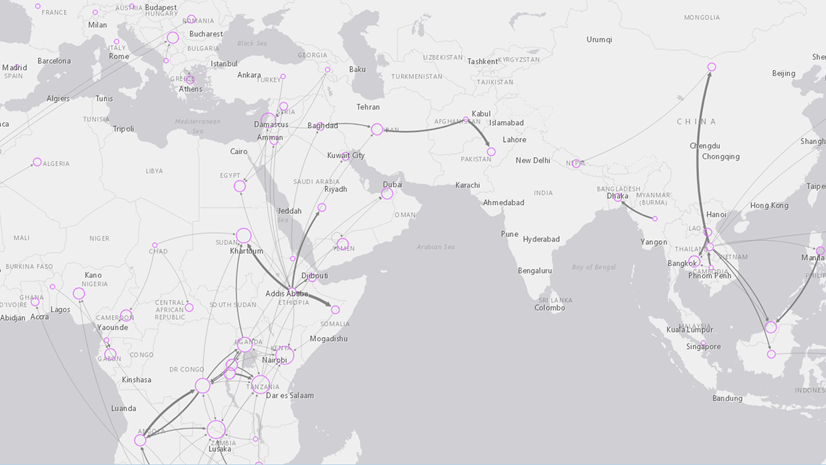ArcMap

# Calculate chainage or distance of points along a polyline

Question: I have a series of polylines with nodes at random points along the line. Is there a way to calculate the chainage distance at each node from the start of the line? Even if a chainage can’t be calculated, is it possible to calculate the distance of each point from the start of the line?

Thanks!

Answer: For this you can use linear referencing. First convert the polylines to routes using the Create Routes tool, and then use the Locate Features Along Routes tool to write the route and measure information to a new event table.

Formerly a Mapping Center Ask a Cartographer  Q & A.Next Article